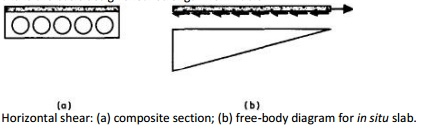Home | | Prestressed Concrete Structures | Concrete Structures: Horizontal Shear

# Concrete Structures: Horizontal ShearThe composite behaviour of the precast beam and in situ slab is only effective if the horizontal shear stresses at the interface between the two regions can be resisted.

HORIZONTAL SHEAR

The composite behaviour of the precast beam and in situ slab is only effective if the horizontal shear stresses at the interface between the two regions can be resisted. For shallow members, such as that shown in Fig. 10.3, there is usually no mechanical key between the two types of concrete, and reliance is made on the friction developed between the contact surfaces. For deeper sections, mechanical shear connectors in the form of links projecting from the beam are used, which provide a much better shear connection. The determination of the horizontal shear resistance is based on the ultimate limit state, and if this condition is satisfied it may be assumed that satisfactory horizontal shear resistance is provided at the serviceability limit state. A simply supported composite section carrying a uniformly distributed load is shown in Fig. 10.8(a) and the free-body diagram for half the length of the in situ slab is shown in Fig. 10.8(b). At the simply supported end there must be zero force in the slab, while the maximum force occurs at the midspan. The distribution of shear forces on the underside of the slab is also shown in Fig. 10.8(b), being zero at midspan and reaching a maximum at the support. This behaviour is similar to that in an elastic beam, where the vertical and horizontal shear stresses increase towards the support for a uniformly distributed load.

The following expression is given in Part 1-3 of EC2 for the horizontal shear stress, where � is the ratio of the longitudinal force in the slab to the total longitudinal force, given by Msd/z, both calculated for a given section; Vsd is the transverse ultimate shear force; z is the lever arm; and bj is the width of the interface.

The design shear resistance for horizontal joints with vertical shear reinforcement is given by

?Rdj=kT?Rd+??N+0.87 fyk? ??0.33 vfck,

where kT is a coefficient with kT=0 if the joint is subjected to tension;

?Rd is the basic design shear strength from TableHorizontal shear: (a) composite section; (b) free-body diagram for in situ slab.

DIFFERENTIAL MOVEMENTS

The fact that the slab of a composite member is usually cast at a much later stage than the beam means that most of the time-dependent effects of shrinkage of the slab take place with the section acting compositely. Most of the shrinkage of the beam will already have occurred by the time the slab is in place, and the movement due to the shrinkage of the slab will induce stresses throughout the whole of the composite section. The water content of the slab concrete is often higher than that of the beam, since a lower strength is required, and this aggravates the problem of differential shrinkage. These extra stresses, which occur even under zero applied load, are not insignificant and should be considered in design. Both the slab and beam undergo creep deformations under load and, although some of the creep deformations in the beam may have taken place before casting of the slab, the level of compressive stress is higher in the beam, and so the creep deformations are larger.Load-deflection curve for composite section in Example 10.4.Differential movements.

composite section which tend to reduce those set up by differential shrinkage. A problem which is encountered, particularly in connection with bridge decks, is that of varying temperature across a composite section, although this may still be a problem in composite members used as roof structures. The hotter upper surface tends to expand more than the cooler lower surface and stresses are induced throughout the composite section.

A method for determining the stresses due to differential shrinkage will now be outlined, and this can be adapted to find the stresses due to differential creep and temperature movements. Consider a composite member as shown in Fig. 10.13, where the slab is shown to have a free shrinkage movement of ?sh relative to the beam. In reality this movement is restrained by the shear forces which are set up between the slab and beam, putting the slab into tension and the beam into compression. The magnitude of the tensile force in the slab is given by

T=?shAc,slabEc,slab,

where Ac,slab and Ec,slab are the cross-sectional area and modulus of elasticity of the slab, respectively, and ?sh is the free shrinkage strain of the slab concrete.

The compressive force in the beam must be numerically equal to this tensile force. In addition to the direct stresses described above, bending stresses are also introduced by restraint of the free differential shrinkage. In order to determine these stresses, the free bodies of the slab and beam are considered, as shown in Fig. 10.14. Initially, the slab can be regarded as having a force T applied through its centroid, so that its length is equal to that of the beam. There must be no net external force on the composite member due to differential shrinkage alone, so a pair of equal and opposite compressive forces must be applied to maintain equilibrium. However, these compressive forces act on the composite section and induce a bending moment at the ends of the member of Internal stress resultants due to differential movements.Stresses due to differential movements.

Study Material, Lecturing Notes, Assignment, Reference, Wiki description explanation, brief detail

Related Topics